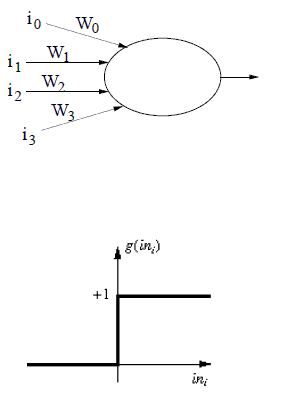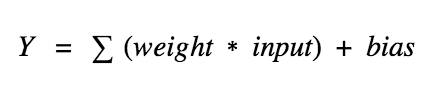2 views

I'm trying to determine for the artificial neuron shown below the values (0 or 1) for the inputs i1, i2, and i3 for which it will fire (i0 is the input for the bias weight and will always be -1).

The weights are

W0 = 1.5

W1 = -1

W2 = 1, and W3 = 2.

Assume the activation function depicted in the image below.

Please clarify your answer as I have done few examples and still I'm not able to fully understand the theory :(

Many thanks,

PS. Image below:by (108k points)

So consider a neuron.Now, the value of Y can be varying from -infinity to +infinity. The neuron actually doesn’t know the bounds of the value. So how do we determine whether the neuron should fire or not ( why this firing pattern? Because we learned it from biology that’s the way brain works and the brain is a working testimony of an awesome and intelligent system ). We will add “activation functions” for this idea. To check the Y value generated by a neuron and decide whether outside connections should consider this neuron as “fired” or not. Or rather let’s say — “activated” or not.

If you wish to learn about artificial neurons then visit this Artificial Neural Network Tutorial.